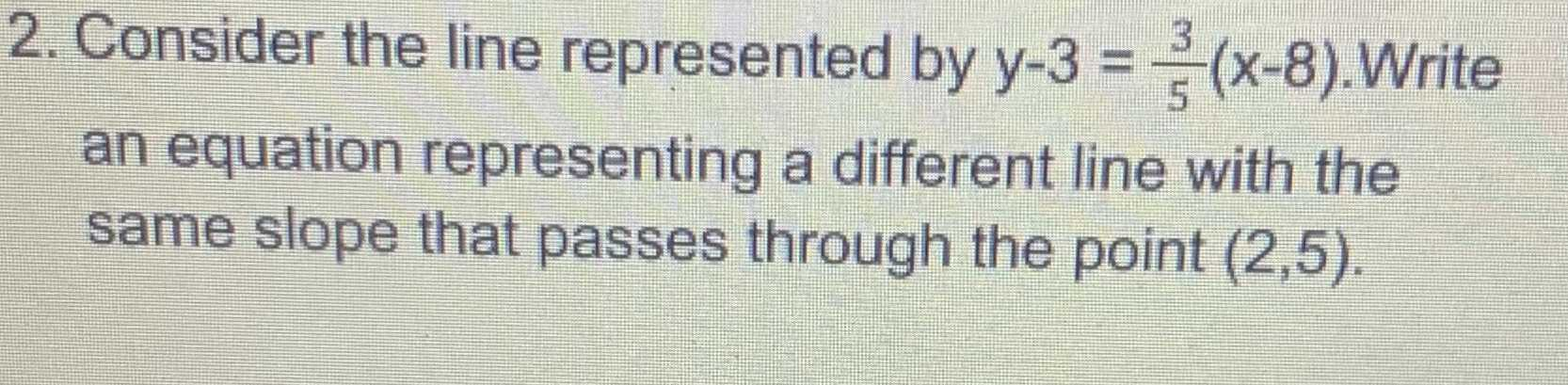### ¿Todavía tienes preguntas de matemáticas?

Pregunte a nuestros tutores expertos
Algebra
Pregunta2. Consider the line represented by $$y - 3 = \frac { 3 } { 5 } ( x - 8 )$$ . Write an equation representing a different line with the same slope that passes through the point $$( 2,5 )$$ .

$$y= \frac{3}{5}x+ \frac{18}{5}$$